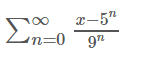# Find the values of x for which the series converges. (Give the answer using interval notation.)

Find the values of x for which the series converges. (Give the answer using interval notation.)